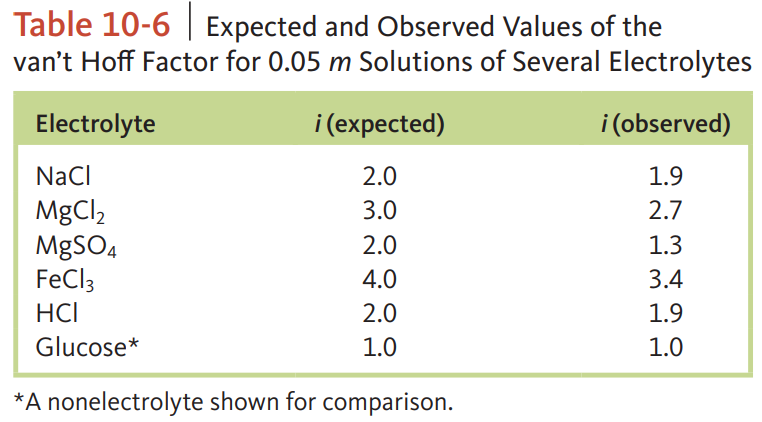Chemistry Practice Problems Freezing Point Depression Practice Problems Solution: Calculate the freezing point and the boiling point...

⚠️Our tutors found the solution shown to be helpful for the problem you're searching for. We don't have the exact solution yet.

# Solution: Calculate the freezing point and the boiling point of a 0.050 m MgCl  2 solution using the observed van’t Hoff factors in Table 10‑6.

###### Problem

Calculate the freezing point and the boiling point of a 0.050 m MgCl  2 solution using the observed van’t Hoff factors in Table 10‑6.Freezing Point Depression

Freezing Point Depression

#### Q. At -12.0°C (a common temperature for household freezers), what is the maximum mass of fructose (C6H12O6) you can add to 1.00 kg of pure water and stil...

Solved • Tue Oct 02 2018 12:15:13 GMT-0400 (EDT)

Freezing Point Depression

#### Q. lf a 0.770 m aqueous solution freezes at -3.30°C, what is the van't Hoff factor, i, of the solute? Kf values can be found here.

Solved • Tue Oct 02 2018 11:08:47 GMT-0400 (EDT)

Freezing Point Depression

#### Q. The following experimental data was collected during a freezing point depression study. Use this data to determine Kf for BHT (butylated hydroxytoluen...

Solved • Tue Oct 02 2018 11:06:43 GMT-0400 (EDT)

Freezing Point Depression

#### Q. Choose the aqueous solution below with the  highest freezing point. These are all solutions of nonvolatile solutes and you should assume ideal van't H...

Solved • Mon Oct 01 2018 15:41:14 GMT-0400 (EDT)